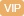| APP | 校园号 | 客服 客服热线：400-863-9889

400-863-9889（2019•吉水县）竖式计算．
308×75=
708÷59=
348÷29=
490÷65=
【考点】整数的乘法及应用整数的除法及应用【专题】计算题．
【分析】先把左边算出得
 4 5
x=.5，利等式的性质，两边时除以
 4 5

【解答】解：x-
 1 5
x0.5
x+2.7=24.7

 4 5
 4 5
=.5÷
 4 5

+0.9×3=24.7
x：
 2 3
=
 3 8
：4
 8 x
=
 2 7

=
 5 8

x22
2x=78
x4=
 1 4
÷4
x÷2=562
4x=
 2 3
×
 3 8

x=28
【点评】此题查运用式的质解方，注意等号要对齐．

0/0